# High School Math : How to find if two acute / obtuse triangles are similar

## Example Questions

### Example Question #1 : How To Find If Two Acute / Obtuse Triangles Are Similar

Are the triangles similar? If so, solve for. (Not drawn to scale).

Yes;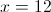Yes;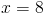Yes;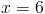The triangles are not similar

Yes;Explanation:

The triangles are similar because of the side-angle-side postulate.

Side: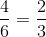Angle: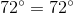Side: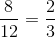The third side must also have a 2:3 ratio.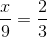Cross-multiply, and solve for.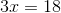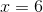### Example Question #2 : How To Find If Two Acute / Obtuse Triangles Are Similar

Are the triangles similar? If so, solve for. (Not drawn to scale).

Yes;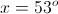Yes;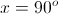The triangles are not similar

Yes;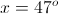The triangles are not similar

Explanation:

The triangles are not similar, as proven by the side-side-side postulate.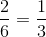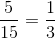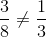The third side does not follow the same ratio of the other two, thus the triangles are not similar.

### Example Question #119 : Triangles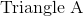and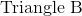are similar triangles.  The perimeter of Triangle A is 45” and the length of two of its sides are 15” and 10”.  If the perimeter of Triangle B is 135” and what are lengths of two of its sides?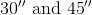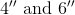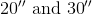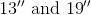Explanation:

The perimeter is equal to the sum of the three sides.  In similar triangles, each side is in proportion to its correlating side.  The perimeters are also in equal proportion.

Perimeter A = 45” and perimeter B = 135”

The proportion of Perimeter A to Perimeter B is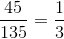This applies to the sides of the triangle.  Therefore to get the any side of Triangle B, just multiply the correlating side by 3.

15” x 3 = 45”

10” x 3 = 30“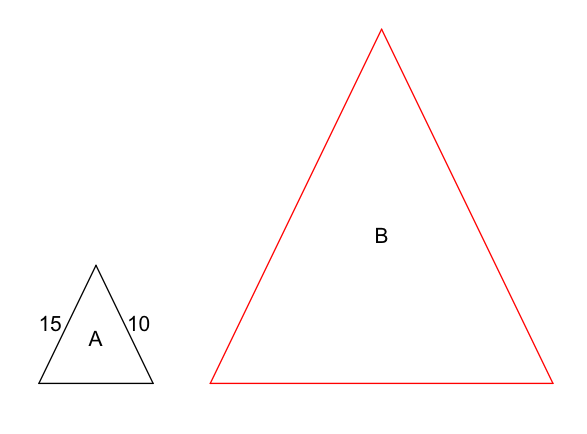### Example Question #3 : How To Find If Two Acute / Obtuse Triangles Are Similar

Side-Side-Angle Postulate

Side-Side-Side Postulate

Side-Angle-Side Postulate

Angle-Angle Postulate

Angle-Angle Postulate

Explanation:

Angle-Angle Postulate: if two angles of one triangle are equal to two corresponding angles of another triangle, the triangles must be similar.

In this example, the triangles share one angle, which must be equal. Additionally, the triangles contain segments that are parallel to each other. When two parallel lines are crossed by another line, the corresponding angles must be equal. Each angle in one triangle is congruent with its corresponding angle in the other triangle, indicating that they are similar.

### Example Question #4 : How To Find If Two Acute / Obtuse Triangles Are Similar

Angle-Angle Postulate

Side-Side-Side Postulate

Side-Angle-Side Postulate

Side-Side-Angle Postulate

Side-Angle-Side Postulate

Explanation:

The Side-Angle-Side Postulate considers two corresponding sides and the included angle. The included angles must be congruent, and the ratios of the two corresponding sides must be equal. If both criteria are satisfied, then the triangles are similar.

In this problem, there is a shared angle, making it equal for the two triangles. Now, consider the ratios of the sides.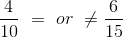Simplify the fractions.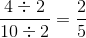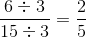because the ratios are equal, the triangles are similar by the Side-Angle-Side postulate.

### Example Question #5 : How To Find If Two Acute / Obtuse Triangles Are Similar

The ratio of the side lengths of a triangle is 7:10:11. In a similar triangle, the middle side is 9 inches long. What is the length of the longest side of the second triangle?

12.1

9

7.7

9.9

10

9.9

Explanation:

Side lengths of similar triangles can be expressed in proportions. Establish a proportion comparing the middle and long sides of your triangles.

10/11 = 9/x

Cross multiply and solve for x.

10x = 99

x = 9.9

### Example Question #185 : Plane Geometry

Two triangles are similar to each other. The bigger one has side lengths of 12, 3, and 14.

The smaller triangle's shortest side is 1 unit in length.  What is the length of the smaller triangle's longest side?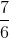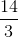Explanation:

Because the triangles are similar, a ratio can be set up between the triangles' longest sides and shortest sides as such: 14/3 = x/1.  Solving for x, we obtain that the shortest side of the triangle is 14/3 units long.

### Example Question #2 : Acute / Obtuse Isosceles Triangles

A triangle with two equal angles is called a(n) __________.

equilateral triangle

right triangle

disjoint triangle

isosceles triangle

Pythagoras triangle